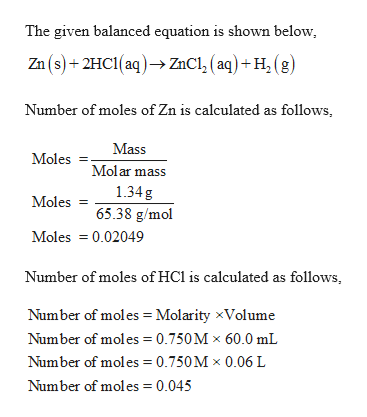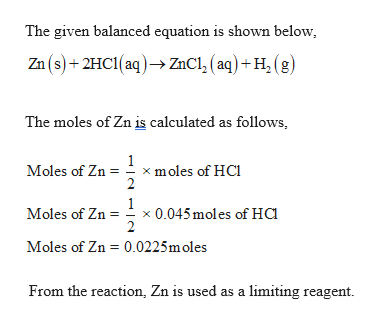# When 1.34 g Zn(s) reacts with 60.0 mL of 0.750 M HCl(aq), 3.14 kJ of heat are produced. Determine the enthalpy change per mole of zinc reacting for the reaction:Zn(?)+2HCl(??)⟶ZnCl2(??)+H2(?)

Question
490 views

When 1.34 g Zn(s) reacts with 60.0 mL of 0.750 M HCl(aq), 3.14 kJ of heat are produced. Determine the enthalpy change per mole of zinc reacting for the reaction:

Zn(?)+2HCl(??)⟶ZnCl2(??)+H2(?)
check_circle

Step 1

Given,

1.34 g Zn reacts with 60.0 mL of 0.750 M HCl(aq).

Heat released is 3.14 KJ.

Molar mass of  Zn = 65.38 g/molhelp_outlineImage TranscriptioncloseThe given balanced equation is shown below Zn(s)+2HC1(aq)ZnCl, (aq)+H (g) Number of moles of Zn is calculated as follows, Mass Moles Molar mass 1.34g Moles 65.38 g/mol Moles 0.02049 Number of moles of HCl is calculated as follows Number of moles = Molarity xVolume Number of moles 0.750 M x 60.0 mL Number of moles= 0.750M x 0.06 L Number of moles 0.045 fullscreen
Step 2

According to the balanced chemical equation, one mole of Zn reacted...help_outlineImage TranscriptioncloseThe given balanced equation is shown below Zn(s)+2HC1(aq)ZnCl, ( aq) +H, (g) The moles of Zn is calculated as follows, 1 x moles of HCl 2 Moles of Zn 1 x 0.045moles of Ha Moles of Zn Moles of Zn 0.0225m oles From the reaction, Zn is used as a limiting reagent fullscreen

### Want to see the full answer?

See Solution

#### Want to see this answer and more?

Solutions are written by subject experts who are available 24/7. Questions are typically answered within 1 hour.*

See Solution
*Response times may vary by subject and question.
Tagged in

### General Chemistry